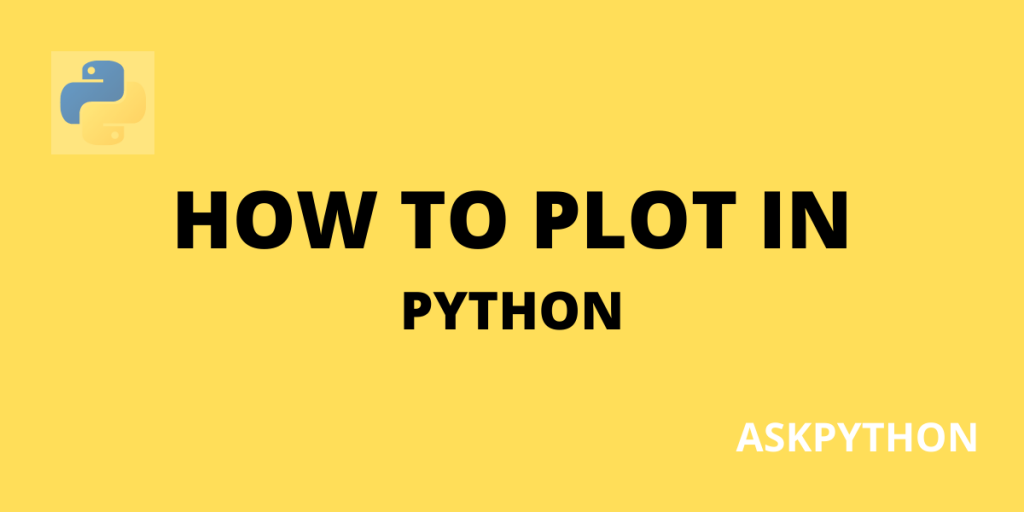# 4 Easy Plotting Libraries for Python With ExamplesPython offers a lot of interactive plotting packages through which we can make some of the most beautiful and customizable graphs and charts available out there. In this article, we will be looking at some of the python modules that are used for plotting and how basic charts are coded with them. These are some of the most widely used python packages and are available for all platforms (like – Windows, Linux Mac).

## 1. Matplotlib – Oldest Plotting Library

If you are accustomed to Python, you must have heard about Matplotlib. It is one of the oldest Python libraries used for plotting, built 18 years ago by Michael Droettboom and originally authored by John D. Hunter, but still remains very popular among python learners and data analysts. It offers an object-oriented application interface that makes matplotlib plots easier to run on a variety of applications.

Let’s look at some codes to plot charts using matplotlib:

### Line Chart

```import matplotlib.pyplot as plt
from numpy import random

var1=random.randint(100, size=(100))
var2=random.randint(100, size=(100))
var1.sort()
var2.sort()

plt.plot(var1,var2)
plt.show()
```

### Histogram

```import matplotlib.pyplot as plt
import numpy as np
from numpy import random
hist_var = np.random.normal(170, 10, 250)
plt.hist(hist_var)
plt.show()
```

## 2. Seaborn

It is a submodule built on matplotlib for creating graphs out of statistical data. Seaborn allows programmers to extract data directly from arrays and data frames and let them visualize a graph out of that statistical data. To allow visualizations, it works under the Matplotlib framework, and for data integration, it relies heavily on pandas.

To understand how seaborn works, we will look into an example code.

### Scatter

```import pandas as pand
from matplotlib import pyplot as plt
import seaborn as sns

def scatter_plot():
sns.lmplot(x='Attack', y='Defense', data=scores,
fit_reg=False,  # It removes a diagonal line that remains by default
hue='Stage'
)
plt.show()

scatter_plot()
```

The code above plots a scatter chart of the attack and defense values we took from the data frame – ‘scores.csv’. The method ‘scatter_plot( )’ contains the seaborn function ‘sns.lmplot’ which plots the scatter by taking ‘Attack’ as the x-axis and ‘Defense’ as y-axis.

Let’s look at another example code. We will be plotting a boxplot by using seaborn, with the same set of values we used in the last example.

### Boxplot

```import pandas as pand
from matplotlib import pyplot as plt
import seaborn as sns

sns.boxplot(data=scores)
plt.show()
```

## 3. Plotly

Plotly is a data visualization tool created in 2012. In this article, we will be learning about a sub-module of Plotly, known as Plotly Express. This sub-module is a Python library with the purpose to create graphic visualizations with a single function call. On the other hand, it also provides a good base to create custom-tailored graphics for media and communication.

Let’s look at a Plotly code example demonstrating how to create simple charts through a single function call.

```import plotly.express as px

def linechart():
df_india = px.data.gapminder().query("country=='India'")
fig = px.line(df_india, x="year", y="lifeExp", title='Average life span in India:')
fig.show()

def scatter():
# x and y given as array_like objects
import plotly.express as px
fig = px.scatter(x=[5, 1, 3, 4, 3], y=[1, 5, 4, 13, 19])
fig.show()

def barplot():
import plotly.express as px
data_Japan = px.data.gapminder().query("country == 'Japan'")
fig = px.bar(data_Japan, x='year', y='pop')
fig.show()

linechart()
barplot()
scatter()
```

In the code above, the program has three different method functions that are called together. Each method function when called plots a chart for the user. If we observe closely, each method function has a different input method. The first function loads data from a Plotly express database. The second function visualizes a scatter chart from values taken from two different arrays. The third function is similar to the first function, it loads data from the Plotly express database and then plots a bar chart.

## 4. Dash

Dash is a Plotly framework that allows us to make web applications and allows us to link graphics, texts, and controls together. This sub-module basically helps in managing various aspects of the application’s frontend such as its layout and styling. The final result is a flask application, which can be easily deployed to various web hosting platforms.

Let’s look at a few of its codes to develop an understanding. The first program plots a life expectancy line chart from the Plotly gapminder database. It plots the life expectancy of all the countries present in the chosen continent.

### Line Chart

```import dash
from dash import dcc
from dash import html
from dash.dependencies import Input, Output
import plotly.express as px

frame_data = px.data.gapminder()
every_continent = frame_data.continent.unique()

app = dash.Dash(__name__)

app.layout = html.Div([
dcc.Checklist(
id="checklist",
options=[{"label": x, "value": x}
for x in every_continent],
value=every_continent[3:],
labelStyle={'display': 'inline-block'}
),
dcc.Graph(id="lineChart"),
])

@app.callback(
Output("lineChart", "figure"),
[Input("checklist", "value")])
def update_line_chart(continents):
x="year", y="lifeExp", color='country')
return figure

app.run_server(debug=True)
```

### Scatter Chart

The code below demonstrates how a scatter chart can be plotted using dash in Python. Here, we used the iris database as our input data frame. The iris database is a pattern recognition dataset containing petal sizes of three different classes of flowers. This program will plot a scatter chart of the petal sizes of the data provided as input.

```import dash
from dash import dcc
from dash import html
from dash.dependencies import Input, Output
import plotly.express as px

frame_data = px.data.iris()

app = dash.Dash(__name__)

app.layout = html.Div([
dcc.Graph(id="plotis_scatter"),
html.P("Width of Petal:"),
dcc.RangeSlider(
id='range-slider',
min=0, max=2.5, step=0.1,
marks={0: '0', 2.5: '2.5'},
value=[0.5, 2]
),
])

@app.callback(
Output("plotis_scatter", "figure"),
[Input("range-slider", "value")])
def update_bar_chart(slider_range):
low, high = slider_range
damask = (frame_data['petal_width'] > low) & (frame_data['petal_width'] < high)
figure = px.scatter(